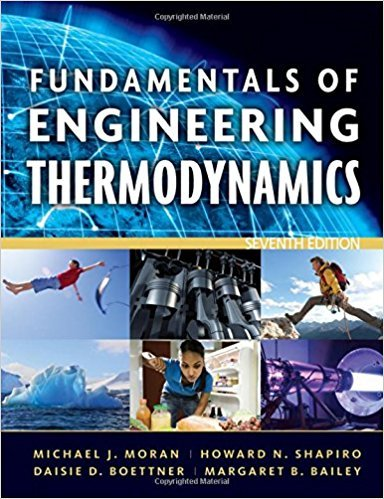×
×

# Evaluating Heat TransferThe outer surface of the grillISBN: 9780470495902 50

## Solution for problem 52P Chapter 2

Fundamentals of Engineering Thermodynamics | 7th Edition

• Textbook Solutions
• 2901 Step-by-step solutions solved by professors and subject experts
• Get 24/7 help from StudySoup virtual teaching assistantsFundamentals of Engineering Thermodynamics | 7th Edition

4 5 1 284 Reviews
27
5
Problem 52P

Evaluating Heat Transfer

The outer surface of the grill hood shown in Fig. P2.52 is at 47°C and the emissivity is 0.93. The heat transfer coefficient for convection between the hood and the surroundings at 27°C is 10 W/m2 · K. Determine the net rate of heat transfer between the grill hood and the surroundings by convection and radiation, in kW per m2 of surface area.Fig. P2.52

Step-by-Step Solution:

Solution 52P

Step 1 of 5</p>

Here we need to calculate the net rate of heat transfer by convection and radiation between the surrounding and grill hood.

The grill hood’s has its outer surface temperature atwith emissivity. The heat coefficient is given to befor the convection heat transfer between the grill hood and surrounding which is at.

Step 2 of 5</p>

Given data,

Outer surface temperature atUsingSurrounding ,UsingEmissivity,Heat coefficient,Stefan Boltzmann Constant,To find,

Rate of heat transfer through convection =?

Rate of heat transfer through radiation =?

Total Net Rate of heat transfer  =?

Step 3 of 5</p>To calculate the rate of heat transfer through convection,

The rate of heat transfer through convection is given by,Substituting,andStep 4 of 5

Step 5 of 5

##### ISBN: 9780470495902

This textbook survival guide was created for the textbook: Fundamentals of Engineering Thermodynamics, edition: 7. Fundamentals of Engineering Thermodynamics was written by and is associated to the ISBN: 9780470495902. The answer to “Evaluating Heat TransferThe outer surface of the grill hood shown in Fig. P2.52 is at 47°C and the emissivity is 0.93. The heat transfer coefficient for convection between the hood and the surroundings at 27°C is 10 W/m2 · K. Determine the net rate of heat transfer between the grill hood and the surroundings by convection and radiation, in kW per m2 of surface area. Fig. P2.52” is broken down into a number of easy to follow steps, and 67 words. Since the solution to 52P from 2 chapter was answered, more than 1083 students have viewed the full step-by-step answer. This full solution covers the following key subjects: transfer, heat, hood, fig, surroundings. This expansive textbook survival guide covers 14 chapters, and 1501 solutions. The full step-by-step solution to problem: 52P from chapter: 2 was answered by , our top Engineering and Tech solution expert on 07/20/17, 09:01AM.

Unlock Textbook Solution